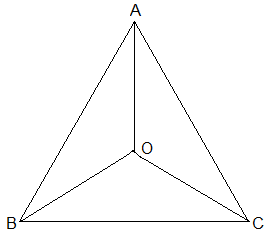Newbie

# In an isosceles triangle ABC, with AB = AC, the bisectors of B and C intersect each other at O. Join A to O. Show that: (i) OB = OC (ii) AO bisects A. Q.1

• 0

Sir please help me to solve the ncert class 9th solution of chapter triangles.How I solve this question of exercise 7.2 question number 1. Find the simplest and easiest solution of this question , also give me the best solution of this question. In an isosceles triangle ABC, with AB = AC, the bisectors of B and C intersect each other at O. Join A to O. Show that: (i) OB = OC (ii) AO bisects A.

Share

1.Solution:

Given:

AB = AC and

the bisectors of B and C intersect each other at O

(i) Since ABC is an isosceles with AB = AC,

B = C

½ B = ½ C

⇒ OBC = OCB (Angle bisectors)

∴ OB = OC (Side opposite to the equal angles are equal.)

(ii) In ΔAOB and ΔAOC,

AB = AC (Given in the question)

AO = AO (Common arm)

OB = OC (As Proved Already)

So, ΔAOB ΔAOC by SSS congruence condition.

BAO = CAO (by CPCT)

Thus, AO bisects A.

• 0